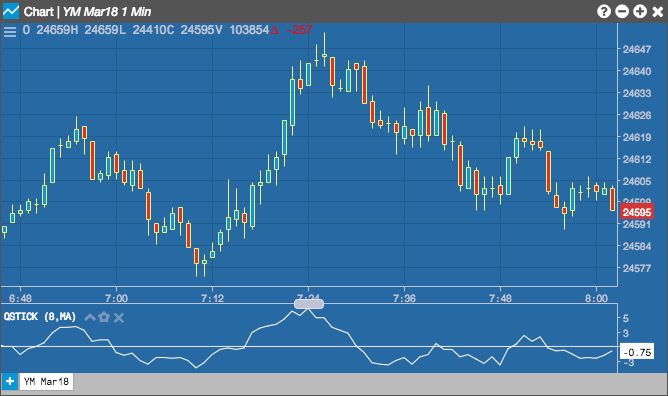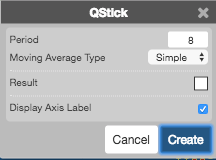Charts

# QStick

The QStick indicator quantifies and identifies trends in candlestick charts. The indicator is a moving average calculation of the difference between the Open and Close prices over a specific period.## Configuration Options• Period: Number of bars to use in the calculations.
• Moving Average Type: Type of moving average to use in the calculations:
• Simple
• Exponential
• Time Series
• Triangular
• Variable
• VIDYA
• Weighted
• Welles Winder
• Hull
• Double Exponential
• Triple Exponential
• Color Selectors: Colors to use for graph elements.
• Display Axis Label: Whether to display the most recent value on the Y axis.

## Formula

$qstick_{t} = \frac{1}{n} \sum_{i=0}^{n-1} \left ( close_{t-i} - open_{t-i} \right )$